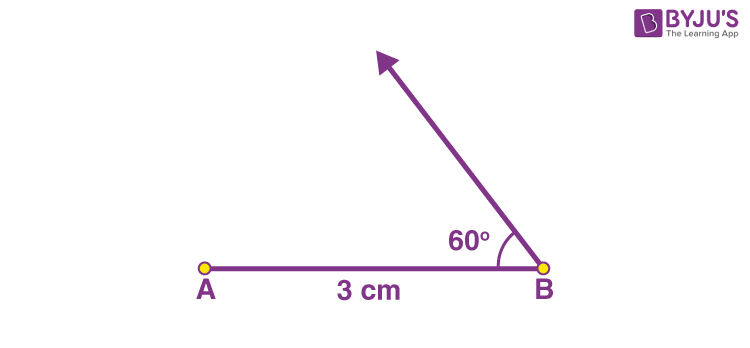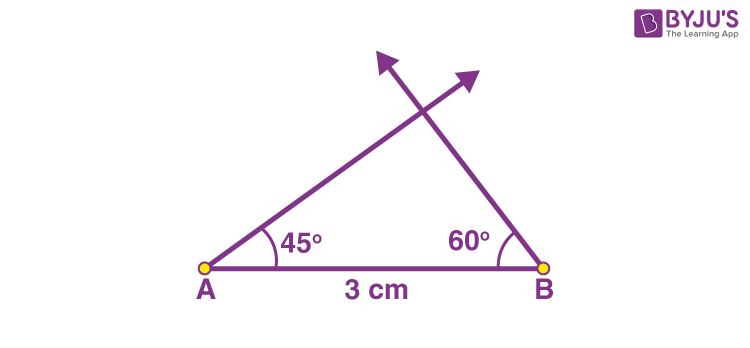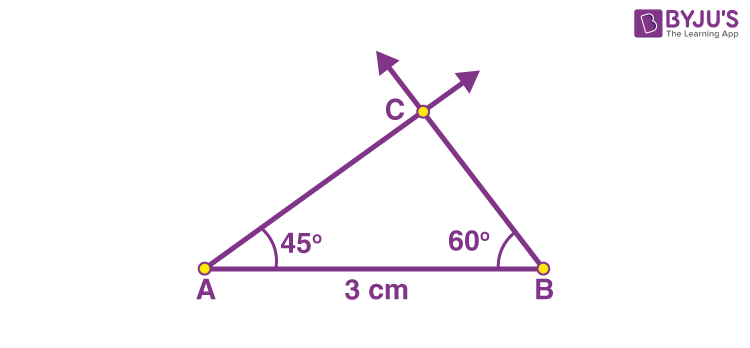Checkout JEE MAINS 2022 Question Paper Analysis : Checkout JEE MAINS 2022 Question Paper Analysis :

# Constructing Triangles, ASA

Constructing ASA triangles explains to construct a triangle where the measure of two angles and one of the side lengths is given to us. Construction of similar triangles is easy because we can easily measure the angles and sides with the help of a ruler and compass. To construct a triangle with provided side length and two angle measurements, you need to follow the below-provided steps. Let us learn here how to construct a triangle when a side length and two angles are given to us.

## ASA Triangle Construction

For the ASA criterion to be satisfied, the given side must necessarily be the one that is enclosed by the known angles.

Now coming back to constructing triangles with the ASA criterion, you will require a ruler and a protractor. Let us say, in a triangle, ABC, the measures of the angles are ∠CAB = 45 degrees and ∠ABC = 60 degrees. The length of side AB = 3cm. The steps for its construction are as follows:

• Step 1: Using the ruler, construct a line segment AB of length 3 cm.• Step 2: Using the protractor, draw a ray at point B making 60 degrees with the line BA.• Step 3: Similarly, draw a ray at point A making 45 degrees with the line AB using the protractor.• Step 4: Mark the point where the two rays meet as C.Thus, you obtain a triangle ABC of the required measurements.

## Video Lesson on Congruency Rules (SAS and ASA)### ASA Triangle Construction – Practice Questions

1. Construct a triangle ABC, whose measurements are given as follows: BC = 6 cm, ∠B = 35° and ∠C = 100°.
2. Construct a triangle XYZ, with base length XY = 8 cm and ∠X = 45° and ∠Y = 65°.
3. Construct a triangle ABC, with AB = 7 units, ∠A = 40°, and ∠B = 105°.

Stay tuned with BYJU’S – The Learning App and download the app to learn all Maths-related concepts easily by exploring more videos.